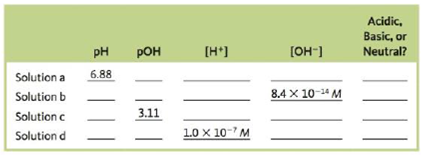# Fill in the missing information in the following table### Chemistry: An Atoms First Approach

2nd Edition
Steven S. Zumdahl + 1 other
Publisher: Cengage Learning
ISBN: 9781305079243

#### Solutions

Chapter
Section### Chemistry: An Atoms First Approach

2nd Edition
Steven S. Zumdahl + 1 other
Publisher: Cengage Learning
ISBN: 9781305079243
Chapter 13, Problem 51E
Textbook Problem
1 views

## Fill in the missing information in the following tableInterpretation Introduction

Interpretation: The missing information in the given table is to be stated.

Concept introduction: The pH is the measure of its [H+] of any solution. The pOH is the measure of the [OH] of any solution. [H+] represents the total hydrogen ion concentration. [OH] represents the total hydroxide ion concentration. The nature of any solution is determined by its pH and pOH value.

To determine: The missing values in the table for solution a.

### Explanation of Solution

Explanation

For solution a

Given

The pH of the solution is 6.88 .

The sum,

pH+pOH=14

Where,

• pOH is the measure of  hyroxide ion concentration.
• pH is the measure of hydrogen ion concentration.

Substitute the value of pH in the above equation.

6.88+pOH=14pOH=146.88pOH=7.12_

The [H+] is 1.31×10-7M_ .

Given

The pH of the solution is 6.88 .

The pH value is calculated by the formula,

pH=log[H+]

Where,

• [H+] is the total concentration of hydrogen ions.

Rearrange the above equation to calculate the value of [H+] .

[H+]=antilog(pH)

Substitute the value of pH in the above equation.

[H+]=antilog(pH)[H+]=Antilog(6.88)[H+]=1.31×10-7M_

The [OH] is 7.6×10-8M_ .

Given

The [H+] is 1.31×107M .

The ionic product of water is,

[H+][OH]=1.0×1014

Where,

• [H+] is the concentration of hydrogen ion.
• [OH] is the concentration of hydroxide ion.

Substitute the value of [H+] in the above equation.

1.31×107×[OH]=1.0×1014[OH]=1.0×10141.31×107[OH]=7.6×10-8M_

The solution is acidic.

In the given solution, the [H+]>[OH] . Therefore, the given solution is acidic.

For solution b

Given

The [OH] is 8.4×1014 .

The ionic product of water is,

[H+][OH]=1.0×1014

Where,

• [H+] is the hydrogen ion concentration.
• [OH] is the hydroxide ion concentration.

Substitute the value of [OH] in the above equation.

[H+]×8.4×1014=1.0×1014[H+]=1.0×10148.4×1014[H+]=0.119M_

The pH is 0.92_

Given

The [H+] is 0.119M

The pH value is calculated by the formula,

pH=log[H+]

Where,

• [H+] is the total concentration of hydrogen ions.

Substitute the value of [H+] in the above equation.

pH=log[0.119]pH=0.92_

The pOH is 13.08_ .

Given

The pH is 0.92 .

The sum, pH+pOH=14

Substitute the value of pH in the above equation.

0.92+pOH=14pOH=140.92pOH=13

### Still sussing out bartleby?

Check out a sample textbook solution.

See a sample solution

#### The Solution to Your Study Problems

Bartleby provides explanations to thousands of textbook problems written by our experts, many with advanced degrees!

Get Started

Find more solutions based on key concepts
Under certain circumstances, amino acids can be converted to glucose and so serve the energy needs of the brain...

Nutrition: Concepts and Controversies - Standalone book (MindTap Course List)

What is the difference between the daily and annual motions of the Sun?

Horizons: Exploring the Universe (MindTap Course List)

How do a solute, a solvent, and a solution differ?

Biology: The Dynamic Science (MindTap Course List)

Write the formulas of ammonium oxide and zinc hydroxide

Introductory Chemistry: An Active Learning Approach

What is meant by residence time? Does seawater itself have a residence time?

Oceanography: An Invitation To Marine Science, Loose-leaf Versin

Two students hold a large bed sheet vertically between them. A third student, who happens to be the star pitche...

Physics for Scientists and Engineers, Technology Update (No access codes included)